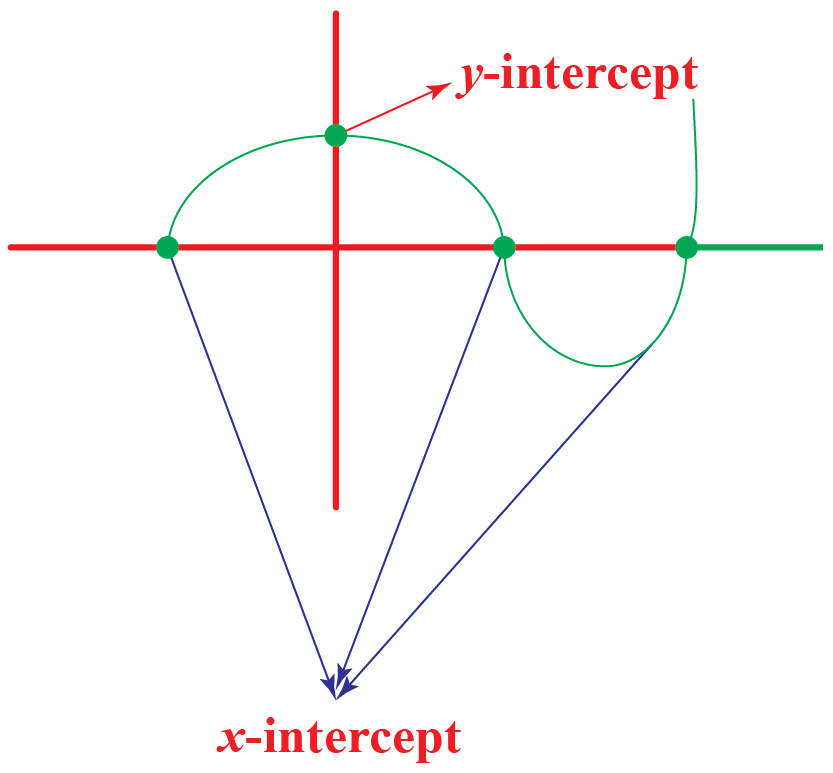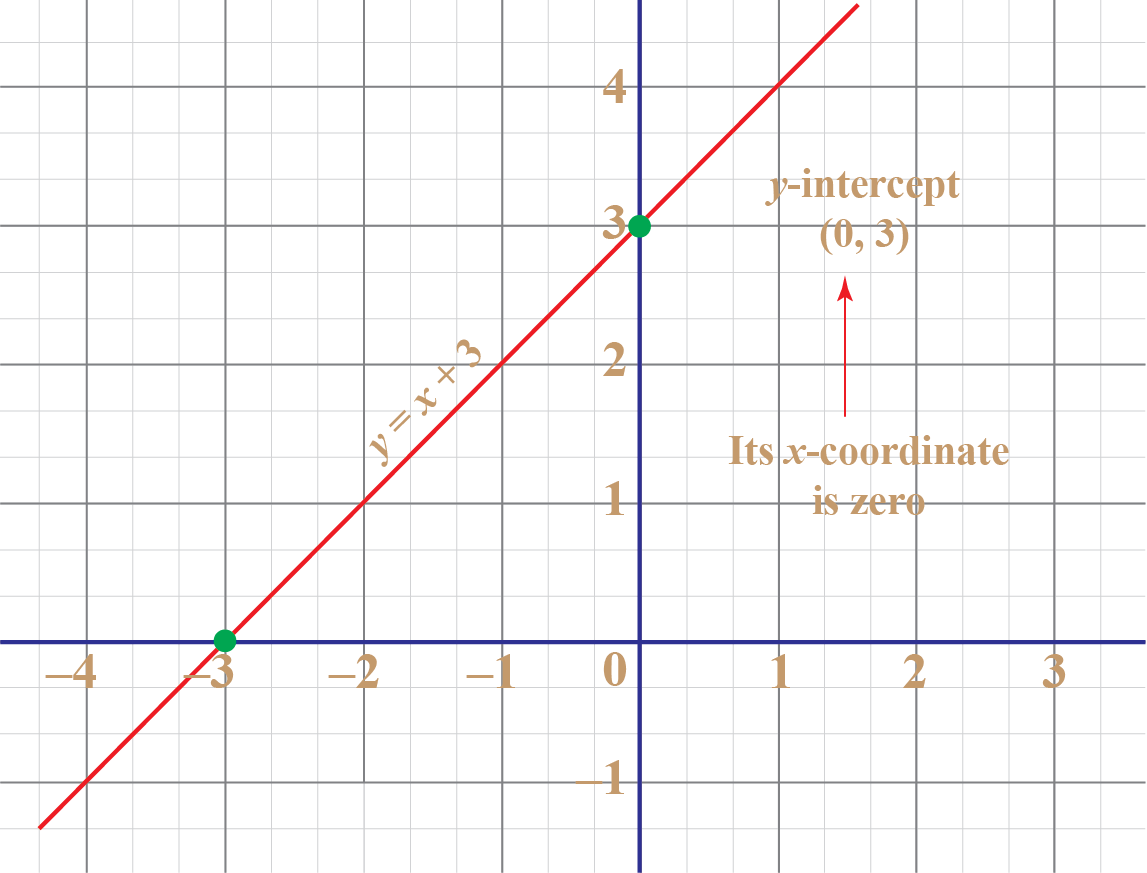# y-intercept

y-intercept

To graph any function that is of the form $$y=f(x)$$ finding the intercepts is really important.

• x-intercept: A point or the points where the graph intersects the x-axis
• y-intercept: A point or the points where the graph intersects the y-axisLet us learn more about y-intercept, the definition of y-intercept and the meaning of y-intercept, definition of y-intercept, example of y-intercept, and explore y-intercept calculator.

Check out the interactive simulations to know more about the lesson and try your hand at solving a few interesting practice questions at the end of the page.

## Lesson Plan

 1 What is y-Intercept? 2 Important Notes on y-Intercept 3 Tips and Tricks 4 Solved Examples on y-Intercept 5 Interactive Questions on y-Intercept

## What is y-Intercept?

### Meaning of y-Intercept

The y-intercept of a graph is (are) the point(s) where the graph intersects the y-axis.

We know that the x-coordinate of any point on the y-axis is 0

So the x-coordinate of a y-intercept is 0

Here is an example of a y-intercept.The y-intercept of the graph is (0, 3) (or) 3 which can be obtained by substituting $$x=0$$ in $$y=x+3$$

Thus, the y-intercept of any graph represented by an equation is obtained by substituting $$x=0$$ in it and solving for $$y.$$

## y-Intercept of a Straight Line

### General Form

The equation of a line in general form is $ax+by+c=0$

For y-intercept, we substitute $$x=0$$ and solve it for $$y.$$

\begin{align} a(0)+by+c &=0\\[0.1cm] by+c&=0\\[0.1cm] y &= \dfrac{-c}{b} \end{align}

Thus, the y-intercept of the equation of a line in general form is:

 $$\left(0, -\dfrac{c}{b} \right)$$ or $$\dfrac{-c}{b}$$

### Slope-Intercept Form

The equation of the line in the slope-intercept form is, $y=mx+b.$

By the definition of the slope-intercept form itself, $$b$$ is the y-intercept of the line.

You try by substituting $$x=0$$ in $$y=mx+b$$ and see whether you get $$b$$ as the y-intercept.

Thus, the y-intercept of the equation of a line in the slope-intercept form is:

 $$\left(0, b \right)$$ or $$b$$

### Point-Slope Form

The equation of the line in the point-slope form is, $y-y_1=m(x-x_1)$

For y-intercept, we substitute $$x=0$$ and solve it for $$y$$

\begin{align} y-y_1 &= m (0-x_1)\\[0.1cm] y-y_1&= -mx_1\\[0.1cm] y&= y_1-mx_1 \end{align}

Thus, the y-intercept of the equation of a line in the point-slope form is:

 $$\left(0, y_1-mx_1 \right)$$ or $$y_1-mx_1$$

## Solved Examples

 Example 1

Can we help James find the y-intercept of the graph of $$y= x+ \dfrac{1}{x}$$?

Solution

To find the y-intercept of the given graph, we substitute $$x=0$$.

Then we get:

$y=0+ \dfrac 1 0 = \infty$

Hence the given graph has no y-intercept. It means the graph does not intersect the y-axis anywhere.

 The y-intercept of the graph does NOT exist
 Example 2

Mia is asked to find the intercepts of the graph represented by the equation $$x=y^{2}+2 y-3$$. Can we help her?

Solution

x-Intercept

To find the x-intercept, we substitute $$y=0$$ in the given equation and solve for $$x$$. Then, $x=0^2+2(0)-3=-3$

y-Intercept

To find the y-intercept, we substitute $$x=0$$ in the given equation and solve for $$y$$. Then,

\begin{align} y^2+2y-3 &=0\\[0.2cm] (y+3)(y-1)&=0\\[0.2cm] y=-3;\;\; &y=1 \end{align}

Thus,

 x-intercept is (-3, 0) y-intercepts are (0, -3) and (0, 1)

## Interactive Questions

Here are a few activities for you to practice.

## Let's Summarize

We hope you enjoyed learning about y-Intercept with the simulations and practice questions. Now, you will be able to easily solve problems on the meaning of y-intercept, definition of y-intercept, example of y-intercept, and y-intercept calculator.

At Cuemath, our team of math experts is dedicated to making learning fun for our favorite readers, the students!

Through an interactive and engaging learning-teaching-learning approach, the teachers explore all angles of a topic.

Be it worksheets, online classes, doubt sessions, or any other form of relation, it’s the logical thinking and smart learning approach that we, at Cuemath, believe in.

## 1. How do you find the y-intercept on the graphing calculator?

We use the button "$$y=$$" on the calculator to enter the function and then press on the "graph."

Then the graph of the function is displayed on the screen.

Then press on the "zoom" button.

Then press on the "trace" button and enter 0

It will then show the value of $$y$$ at which $$x$$ is 0

## 2. What is the y-intercept of $$y= 3x?$$

To find the y-intercept, substitute $$x=0$$

Then $y=3(0)=0$

So the y-intercept of $$y=3x$$ is $$(0,0)$$

## 3. What is the slope of $$y = 6?$$

Comparing $$y=6$$ with $$y=mx+b$$, we get, $m=0\\b=6$

Here, $$m$$ is the slope of the line.

Thus, the slope of $$y=6$$ is 0

More Important Topics
Numbers
Algebra
Geometry
Measurement
Money
Data
Trigonometry
Calculus